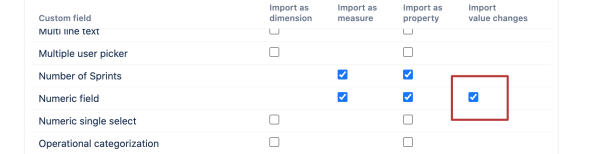# Count property value by the date it was filled

Hi,
I wish to count property field and present it on a report by date issue was closed\last updates or property become not empty.

The below calculated measure not working.
using [Measures].[Issues created] > 0 counting it on the day the issues was created, which is not what we are looking for.

NonZero(
sum(
Filter(
Descendants([Issue].CurrentMember,[Issue].[Issue]),
Not IsEmpty([Measures].[Issue Cost Reduction]) --custom field imported as property and filter
AND
–measure to add context of the report
[Measures].[Issues closed] > 0 )
)

Hi,

Numeric custom fields can be imported into eazyBI with the change history:After the import, you should have a measure giving the history of this field.
You can create the following custom formula for finding the value of the measure on a specific date, for instance, issue closing date:

``````([Measures].[Numeric field history],
[Time].[Day].DateMember([Measures].[Issue closed date]))
``````

With a similar approach you can find the initial value of the numeric field:

``````([Measures].[Numeric field history],
Filter(
[Time].[Day].Members,
[Measures].[Numeric field history]>0
).item(0))
``````

Kindly,
Janis, eazyBI support